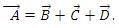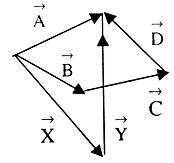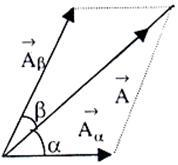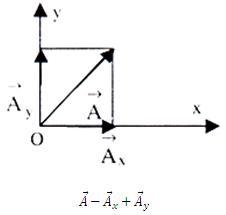Click to Chat

1800-1023-196

+91-120-4616500

CART 0

• 0

MY CART (5)

Use Coupon: CART20 and get 20% off on all online Study Material

ITEM
DETAILS
MRP
DISCOUNT
FINAL PRICE
Total Price: Rs.

There are no items in this cart.
Continue Shopping• Kinematics & Rotational Motion
• OFFERED PRICE: Rs. 636
• View Details

```Vector Components

Components of a Vector:
From the figure given below we can writeIt means B,C  and D vectors are the components of vector A.

Note that we can also write A =X +Y. So vectors X and Y are also components of vector A. It implies that we can draw any number of set of components in any desired direction.

For resolving a vector A along two directions making angles α and with it as shown in figure given below, we use the following:-Aα = (A sinβ)/(sin(α + β))

Perpendicular Components

Representation of any vector lying in the x – y plane, as shown in figure given below, as the sum of two vectors, one parallel to the x-axis and the other parallel to y-axis, is extremely useful in physical analysis because both have mutually independent effects. These two vectors are labeled A and A. These are called the perpendicular or rectangular component of vector A and are expressed as:If magnitude and direction of vector A are known then Ax = A cosθ and Ay = A sinθ. Hence we can write

Ax2 + Ay2 = A2 and tanθ = Ay/Ax

Now, it should be clear that if we have to add 30 vectors, we will resolve each of these 30 vectors in rectangular components in any x and y direction. Then simply add all the components in the x direction and all the components in the y direction. Adding these two resultant perpendicular components will give us the final resultant.

Illustration:

A vector quantity of magnitude L acts on a point A along the direction making an angle of 450 with the vertical, as shown in the figure given below. Find the component of this vector in the vertical direction?Solution:

The component of the vector in the vertical direction will be

L*(cos ∏/4) = L/√2.

Learning components of a vector is very important from IIT JEE, AIEEE and other engineering exams perspective. Most of the Physics is based on usage of vectors and components of vectors. Vectors and their components are also useful in trigonometry and mathematics as a whole. This is a very useful concept and should be learned thoroughly at the beginner’s level so that it paves a way for great understanding of statistics, physics and mathematics at higher levels.

AskIITians.com provides free study material for students aspiring for IIT JEE AIEEE and other engineering entrance exams. We also provide live online IIT JEE and AIEEE preparation over internet. You can study in our live online batches and do your exam preparation with us from your home. Utilize askIITians free resources like:- FREE Study Material, FREE A Question A Day, FREE Test Series while preparing for IIT JEE, AIEEE and other engineering entrance exams.

To read more, Buy study materials of Units & Dimensions comprising study notes, revision notes, video lectures, and previous year solved questions etc. Also browse for more study materials on Physics here
```### Course Features

• 731 Video Lectures
• Revision Notes
• Previous Year Papers
• Mind Map
• Study Planner
• NCERT Solutions
• Discussion Forum
• Test paper with Video Solution